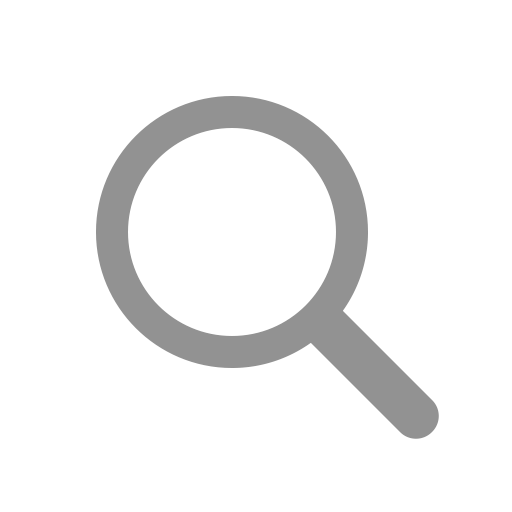Singapore Airport Guide to Singapore Changi Airport (SIN)

# Airlines at Singapore Changi Airport (SIN)

## Airlines operating at Singapore Changi Airport (SIN):

List of airlines operating at Singapore Changi Airport (SIN). Airline code and more informationIATA Code: A3
+
IATA Code: KC
+
Terminal: 3
IATA Code: AC
+
Terminal: 1
IATA Code: CA
+
IATA Code: UX
+
Terminal: 1
IATA Code: AF
+
Terminal: 2
IATA Code: AI
+
Terminal: 2
IATA Code: IX
+
IATA Code: IG
+
Terminal: 1
IATA Code: MK
+
Terminal: 3
IATA Code: NZ
+
Terminal: 1
IATA Code: PX
+
IATA Code: JU
+
Terminal: 4
IATA Code: AK
+
Terminal: 4
IATA Code: D7
+
IATA Code: AZ
+
Terminal: 2
IATA Code: NH
+
IATA Code: AA
+
Terminal: 3
IATA Code: OZ
+
IATA Code: OS
+
IATA Code: AV
+
Terminal: 1
IATA Code: PG
+
Terminal: 3
IATA Code: ID
+
Terminal: 1
IATA Code: BG
+
Terminal: 1
IATA Code: BA
+
IATA Code: SN
+
IATA Code: K6
+
Terminal: 4
IATA Code: CX
+
Terminal: 4
IATA Code: 5J
+
Terminal: 3
IATA Code: CI
+
Terminal: 3
IATA Code: MU
+
Terminal: 1
IATA Code: CZ
+
Terminal: 1
IATA Code: OQ
+
IATA Code: OU
+
Terminal: 1
IATA Code: DL
+
Terminal: 1
IATA Code: KB
+
IATA Code: MS
+
Terminal: 1
IATA Code: EK
+
Terminal: 2
IATA Code: ET
+
Terminal: 2
IATA Code: EY
+
Terminal: 3
IATA Code: BR
+
Terminal: 1
IATA Code: FJ
+
Terminal: 1
IATA Code: AY
+
IATA Code: FY
+
IATA Code: XY
+
Terminal: 3
IATA Code: GA
+
Terminal: 4
IATA Code: G8
+
Terminal: 4
IATA Code: GX
+
IATA Code: GF
+
Terminal: 4
IATA Code: HU
+
IATA Code: HA
+
Terminal: 1
IATA Code: NS
+
IATA Code: IB
+
Terminal: 2
IATA Code: 6E
+
Terminal: 4
IATA Code: QZ
+
Terminal: 1
IATA Code: JL
+
Terminal: 4
IATA Code: 7C
+
Terminal: 1
IATA Code: JQ
+
Arrivals Terminal: 1 or 2
Departures Terminal: 1
IATA Code: 3K
+
Terminal: 1
IATA Code: BL
+
Terminal: 4
IATA Code: HO
+
Terminal: 1
IATA Code: KL
+
Terminal: 4
IATA Code: KE
+
IATA Code: LA
+
Terminal: 3
IATA Code: JT
+
Terminal: 2
IATA Code: LO
+
Terminal: 2
IATA Code: LH
+
Terminal: 2
IATA Code: MH
+
Terminal: 3
IATA Code: OD
+
IATA Code: OM
+
Terminal: 1
IATA Code: 8M
+
Terminal: 3
IATA Code: UB
+
IATA Code: WY
+
Terminal: 1
IATA Code: PR
+
Terminal: 4
IATA Code: Z2
+
Terminal: 1
IATA Code: QF
+
Terminal: 1
IATA Code: QR
+
Terminal: 4
IATA Code: RX
+
IATA Code: AT
+
Terminal: 2
IATA Code: BI
+
IATA Code: RJ
+
IATA Code: S7
+
IATA Code: SK
+
Terminal: 3
IATA Code: SV
+
Terminal: 1
IATA Code: TR
+
Terminal: 1
IATA Code: ZH
+
Terminal: 2
IATA Code: 3U
+
Terminal: 2
IATA Code: MI
+
Terminal: 2 or 3
IATA Code: SQ
+
IATA Code: SA
+
Terminal: 4
IATA Code: 9C
+
Terminal: 3
IATA Code: UL
+
Terminal: 2
IATA Code: LX
+
IATA Code: TP
+
Terminal: 4
IATA Code: FD
+
Terminal: 1
IATA Code: TG
+
Terminal: 3
IATA Code: SL
+
Terminal: 1
IATA Code: TK
+
Terminal: 2
IATA Code: UA
+
Terminal: 4
IATA Code: UQ
+
Terminal: 3
IATA Code: BS
+
Terminal: 4
IATA Code: VJ
+
Terminal: 4
IATA Code: VN
+
IATA Code: VS
+
IATA Code: VA
+
Terminal: 3
IATA Code: UK
+
Terminal: 1
IATA Code: MF
+